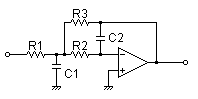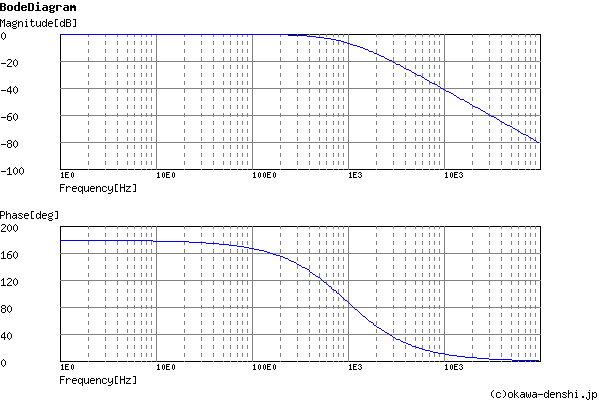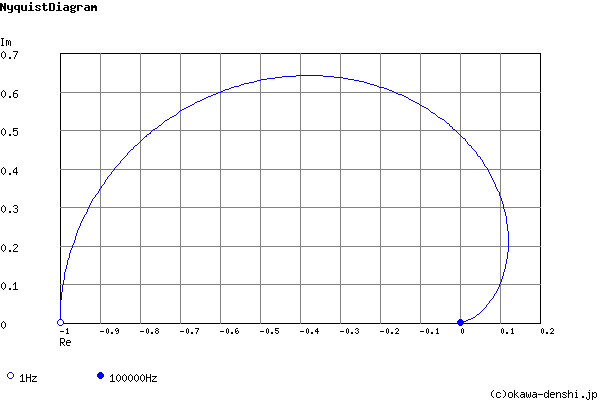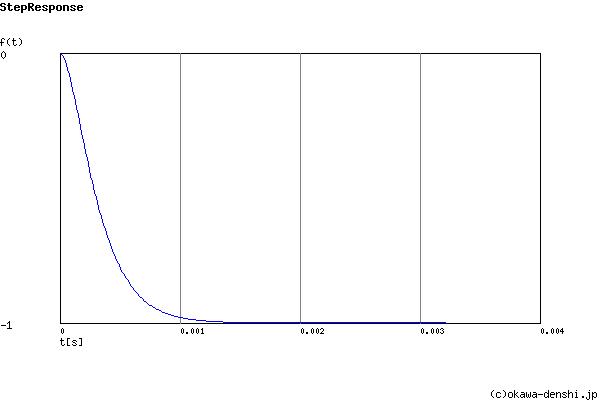# (Sample)Multiple Feedback Low-pass Filter Design Tool - Result -

Calculated the Transfer Function for the multiple feedback low-pass filter, displayed on graphs, showing Bode diagram, Nyquist diagram, Impulse response and Step response.

## Multiple Feedback Filter

 Vin(s)→→Vout(s)
(Sample)Transfer Function:
 G(s)= -39313739.3656 s2+12601.6260163s+39313739.3656

R1 = 8.2kΩ
R2 = 30kΩ
R3 = 8.2kΩ
C1 = 0.022uF
C2 = 0.0047uF

#### Cut-off frequency

fc = 997.912146175[Hz]

#### Gain at f=0Hz

Gpk = -1[times] (0)[dB]

#### Quality factor

Q = 0.497560150302

#### Damping ratio

ζ = 1.00490362762

#### Pole(s)

p = -903.859636226[Hz]
|p|= 903.859636226[Hz]
p = -1101.75143526[Hz]
|p|= 1101.75143526[Hz]

#### Phase margin

pm= NAN[deg] (f =0[Hz])

#### Overshoot (in absolute value)

The peak of transient waveform is not detected.

#### Final value of the step response (on the condition that the system converged when t goes to infinity)

g(∞) = -1

fc=Hz
Gain K= at f=0Hz. (K<0)

### Q factor | Damping ratio ζ

Quality factor Q =
Damping ratio ζ =
 C1 = F C2 = F
C1, C2 is optional. But when setting these capacitances, C1 and C2 of both are needed to give following the equation
(C2/C1)≤ζ 2/(1-K)
(C1/C2)≥Q2(1-K)

Select Capacitor Sequence:
Select Resistor Sequence:

### Frequency analysis

Bode diagram
Phase  Group delay
Nyquist diagram
Pole, zero
Phase margin
Oscillation analysis
Analysis on frequency range:
f1=∼f2=[Hz] (optional)

### Transient analysis

Step response
Impulse response
Overshoot
Final value of the step response
Analysis on time range:
0∼[sec] (optional)

# Frequency analysis# Transient analysis